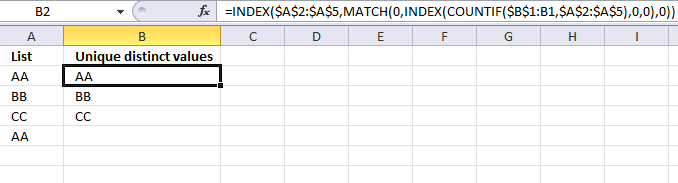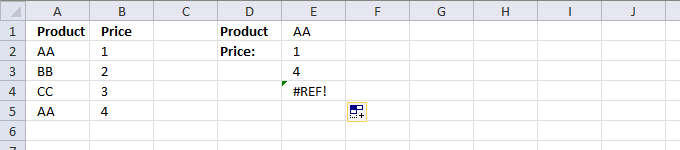Author: Oscar Cronquist Article last updated on July 08, 2018

Kamran Mumtaz asked: Is there any way to use VLOOKUP for multiple criteria and I do not want to use CSE?;

My answer: No, not to my knowledge

Kamran Mumtaz: This is the formula given by Aladin Akyurek without (CSE)...

=INDEX(Sheet3!\$B\$2:\$B\$65, MATCH(1,INDEX((Sheet3!\$C\$2:\$C\$65=E\$1)*(Sheet3!\$A\$2:\$A\$65=\$A3), 0, 1), 0))

----------------------------------------------------------------------------------------------------------------------------

This formula is really interesting, I have never seen this approach before. It definitely looks like an array formula but it is not. It opens up possibilities that I have not thought about before.

Example 1,This formula derives from one of the most popular blog posts here: Extract a unique distinct list

The original array formula:

=INDEX(\$A\$2:\$A\$5,MATCH(0,COUNTIF(\$B\$1:B1,\$A\$2:\$A\$5),0))

The new array formula in cell B2:

=INDEX(\$A\$2:\$A\$5,MATCH(0,INDEX(COUNTIF(\$B\$1:B1,\$A\$2:\$A\$5),0,0),0))

The new formula is NOT an array formula! Amazing! I am not sure if all array formulas can be converted to regular formulas but obviously some can.

Example 2,This formula derives from one of the most popular blog posts here: How to return multiple values using vlookup

Original array formula:

=INDEX(\$B\$2:\$B\$5, SMALL(IF(\$E\$1=\$A\$2:\$A\$5, MATCH(ROW(\$B\$2:\$B\$5),ROW(\$B\$2:\$B\$5)), ""), ROW(A1)))

New array formula in cell E2:

=INDEX(\$B\$2:\$B\$5, SMALL(INDEX((\$A\$2:\$A\$5=\$E\$1)*(MATCH(ROW(\$B\$2:\$B\$5), ROW(\$B\$2:\$B\$5)))+(\$A\$2:\$A\$5<>\$E\$1)*1048577, 0, 0), ROW(A1)))